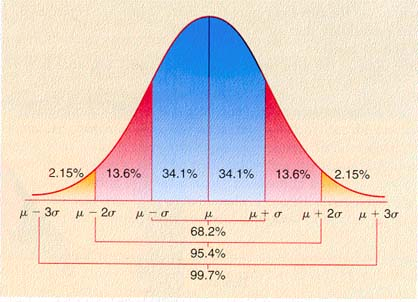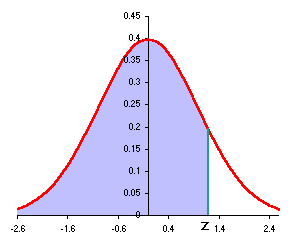Statistic or Parameter Symbol Equations Excel
Basic Statistics
Square root Ö =SQRT(number)
Sample size n =COUNT(data)
Sample mean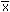Sx/n =AVERAGE(data)
Population mean µ SX/N =AVERAGE(populationData)
Sample standard deviation sx or s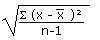=STDEV(data)
Sample Coefficient of Variation CV 100(sx/) =100*STDEV(data)/AVERAGE(data)
Linear Regression Statistics
Slope b =SLOPE(y data, x data)
Intercept a =INTERCEPT(y data, x data)
Correlation r =CORREL(y data, x data)
Coefficient of Determination =(CORREL(y data, x data))^2
Normal Statistics
Calculate a z value from an x z =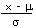=STANDARDIZE(x, m, s)
Calculate an x value from a z x = s z + m = s*z+m
Calculate a z-statistic from an x z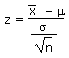=(-µ)/(sx/SQRT(n))
Calculate a t-statistic (tstat) t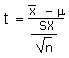=(-µ)/(sx/SQRT(n))
Calculate an x from a z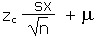=m + zc*sx/sqrt(n)
Find a probability p from a z value =NORMSDIST(z)
Find a z value from a probability p =NORMSINV(p)
Confidence interval statistics
Degrees of freedom df = n-1
Find a zc value from a confidence level c zc =ABS(NORMSINV((1-c)/2))
Find a tc value from a confidence level c tc =TINV(1-c,df)
Calculate an error tolerance E of a mean for n ³ 30 using sx E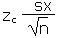=zc*sx/SQRT(n)
Calculate an error tolerance E of a mean for n < 30 using sx. Can also be used for n ³ 30. E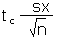=tc*sx/SQRT(n)
Calculate a confidence interval for a population mean m from a sample mean x and an error tolerance E x-E< m <x+E
Hypothesis Testing
Calculate t-critical for a two-tailed test tc =TINV(a,df)
Calculate t-critical for a one-tailed test tc =TINV(2*a,df)
Calculate a p-value from a t-statistic p = TDIST(ABS(tstat),df,#tails)